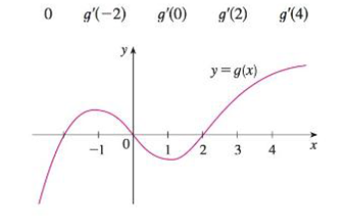Chapter 2.7, Problem 17E### Single Variable Calculus: Early Tr...

8th Edition
James Stewart
ISBN: 9781305270343

#### Solutions

Chapter
Section### Single Variable Calculus: Early Tr...

8th Edition
James Stewart
ISBN: 9781305270343
Textbook Problem

# For the function g whose graph is given, arrange the following numbers in increasing order and explain your reasoning:To determine

To find: The arrange the following numbers in increasing order and reasoning.

Explanation

Result used:

The slope of the line passing through the points (x1,y1) and (x2,y2) is y2y1x2x1.

Calculation:

Obtain the values g(2).

From the given graph, draw the tangent line at x=2 is approximately joining the points (2,0) and (2.2,0.3).

Use the slope formula stated above and compute the slope of the line passing through the points (2,0) and (2.2,0.3).

g(2)=0.302.2+2=0.30.2=0.30.2=1.5

Thus, the value is g(2)=1.5.

Obtain the values g(0).

From the given graph, draw the tangent line at x=0 is approximately joining the points (0,0) and (0.1,0.1).

Use the slope formula stated above and compute the slope of the line passing through the points (0,0) and (0.1,0.1).

g(0)=0.100.1+0=0.10.1=1

Thus, the value is g(0)=1.

Obtain the values g(2)

### Still sussing out bartleby?

Check out a sample textbook solution.

See a sample solution

#### The Solution to Your Study Problems

Bartleby provides explanations to thousands of textbook problems written by our experts, many with advanced degrees!

Get Started

#### Find the mean for the following set of scores: 2, 7, 9, 4, 5, 3, 0, 6

Essentials of Statistics for The Behavioral Sciences (MindTap Course List)

#### Evaluate the integral. 30. 12|ex1|dx

Single Variable Calculus: Early Transcendentals

#### The graph of is: a) b) c) d)

Study Guide for Stewart's Single Variable Calculus: Early Transcendentals, 8th

#### Find for .

Study Guide for Stewart's Multivariable Calculus, 8th